×#### Thank you for registering.

One of our academic counsellors will contact you within 1 working day.

Click to Chat

1800-1023-196

+91-120-4616500

CART 0

• 0

MY CART (5)

Use Coupon: CART20 and get 20% off on all online Study Material

ITEM
DETAILS
MRP
DISCOUNT
FINAL PRICE
Total Price: Rs.

There are no items in this cart.
Continue Shopping```Working Rule for finding the Area

(i) If curve lies completely above the x axis, then the area is positive but when it lies completely below x axis, then the area is negative, however we have the convention to consider the magnitude only.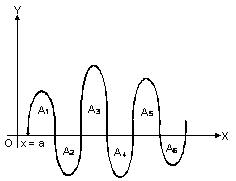(ii) If curve lies on both the sides of x axis i.e. above the x axis as well as below the x axis, then calculate both areas separately and add their modulus to get the total area.

In general if curve y = f(x) crosses the x axis n times when x varies from a to b, then the area between y = f(x), x axis and lines x = a and x = b is given by

A = |A1| + |A2| + ... + |A11|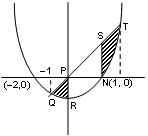(iii) If the curve is symmetrical about x axis, or y axis, or both, then calculate the area of one symmetrical part and multiply it by the number of symmetrical parts to get the whole area.

Illustration 15  Find the area between the curves y = x2 + x –2 and y = 2x, for which |x2+ x –2| + | 2x | = |x2 + 3x –2| is satisfied.

Solution:

y = x2 + x - 2 => y = 2x

|x2 + x - 2| + |2x| = |x2 + 3x -2|

(x2 + x - 2) and 2x have same sign

Thus required area

ar (PQR) + ar (ECD)

=  ∫0–1 [2x - (x2 + x - 2)] dx + ∫21 [2x - (x2 + x - 2)] dx

= [x2/2 – x3/3 + 2x]0–1 + [x2/2 – x3/3 + 2x]21

= 7/6 + [10/3 –13/6] = 7/6 + 7/6 = 7/3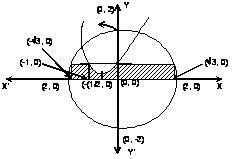Illustration 16  Find out the area enclosed by circle |z| = 2, parabola y = x2 + x + 1, the curve y = [sin2 x/4 + cos x/4] and x-axis (when [ . ] is the greatest integer function).

Solution:

For x ∈ [-2, 2]

=> 1 < sin2 x/4 + cos x/4 < 2

∴ [sin2 x/4 + cos x/4] = 1

Now we have to find out the area enclosed by the circle |z| = 2,

parabola (y - 3/4) = (x+1/2)2,

line y = 1and x-axis.

∴ Required area is shaded area in the figure.

Hence required area = √3 × 1 + (√3 – 1) +  ∫01 (x2 + x + 1).dx + 2  ∫2√3√4–x2 dx

= (2π/3 + √3 – 1/6) sq. units

Illustration 17:  Let f(x) = Max. {sinx, cos x, 1/2} then determine the area of the region bounded by the curves y = f(x), x-axis, y-axis and x = 2π.

Solution:

f(x) = Max {sin x, cos x, 1/2}

interval value of f(x)

for 0 < x < π/4, cos x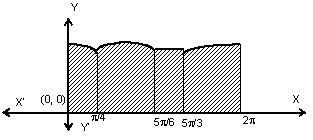for  π/4 < x < 5π/6,  sin x

for 5π/6 < x < 5π/3, 1/2

for   5π/3 < x < 2π       cos x

Hence required area

= ∫π/40 cosx dx + ∫5π/6π/4 sin x dx + ∫5π/35π/6 1/2.dx + ∫2π5π/3 cosx dx

= (5π/12 + √2 + √3) sq units.

Illustration 18: Find area bounded by y = |4 – x2|/4 and y = 7 - |x|

Solution:

y = |4 – x2|/4        (I)

y = 7 – |x|       (II)

Rewriting (I) and (II)

y = {4–x2 / 4 – 2< x < 2

x2–4/4 x∈(–∞, –2) U (2, ∞)

y = {7 – x     x >0     7 + x    x < 0

Required area (PQRSTUP) = 2 area         (PQRSP)

= 2 [area of (OVRSO) – area of (POQP) –         area of (QRVQ) ]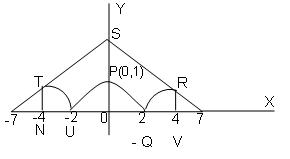= 2 [1/2 (7 + 3)4 - ∫20 (4–x2/4) dx – ∫42 x2–4/4 dx]

= 2 18 + 2/3 – 16/3 + 4 + 2/3 – 2

= 32 sq unit.

Illustration 19: Let f be a real valued function satisfying f(x/y) and limx–>0 f(1+x)/x = 3. Find the area bounded by the curve y = f(x), the y axis and the line y = 3

Solution:

Given = f(x/y) = f(x) – f(y)                                                                        .... (1)

Putting x = y = 1, we get f(1) = 0

Now,    f’(x) = limh–>0 f(x+h) – f(x) / h

= Limh–>0 f(1+h/x)/h         (from(1))

= Limh–>0 f(1+h/x) (h/x)x

=>   f'(x) = 3/x  {since Limx–>0 f(1+x0/x = 3}

=>   f(x) = 3 ln x + c

Putting x = 1

=>   c = 0

=>   f(x) = 3 ln x = y (say)

Required area = ∫3–∞ x dy = ∫3–∞ ey/3 dy  = 3[ey/3]3–∞

= 3 (e – 0) = 3e sq. units

Illustration 20:   Let An be the area bounded by y = tann x, x =0, y = 0 and x = p/4. Prove that for n ³ 2.   (i) An+An-2 = 1/n–1  (ii) 1/2(n+1) < An < 1/2(n–1)

Solution:  (i)  Obviously An = .∫π/40 tann xdx

An+An–2 = ∫π/40 (tann xdx + tann–2x)dx   =∫10 tn–2 dt = 1/n–1.

(ii) Obviously An+2< An< An-2    (from figure).

Thus 2 An = An + An < An + An-2 = 1/n–1 (by part (i)

Thus An < 1/2(n–1)              . . . . (1)

Also 2 An = An+ An > An + An+2 = 1/n+1, replacing n by n+2 in (i)

An > 1/2(n+1)                      . . . . (2)

From (1) and (2), we get 1/2(n+1) < An < 1/(2n–1).

To read more, Buy study materials of Definite integral comprising study notes, revision notes, video lectures, previous year solved questions etc. Also browse for more study materials on Mathematics here.
```### Course Features

• 731 Video Lectures
• Revision Notes
• Previous Year Papers
• Mind Map
• Study Planner
• NCERT Solutions
• Discussion Forum
• Test paper with Video Solution